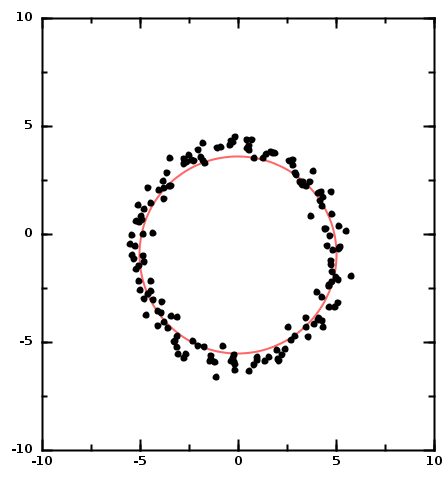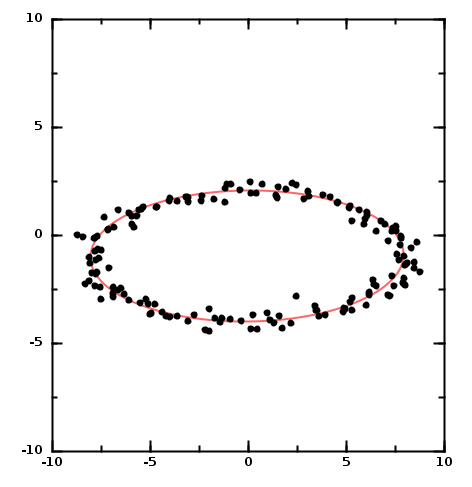# DMelt:DataAnalysis/7 Fitting Shapes

Limitted access. First login to DataMelt if you are a full DataMelt member. Then login to HandWiki as a user.

# Fitting data with shapes

DataMelt offers classes to fit 2D data using shapes. Typically, shapes can be specified using equations (for example, parametric). In this section we will show how to fit data using ellipses and circles.

## Fitting data with circles

Let us perform a least-squires minimisation of points with the goal to determine a circle that best fits the collection of points. The fit determines the circle position and radius. In addition, it calculates how well points distributed around the circle. In this approach we use parametric equations.

Here is a visual example:This plot was made using the following script:

## Fitting data with ellipses

This approach also uses the algorithm of Fitzgibbon et al and by Halir et al, to find the ellipse which best approximates a collection of points.

As before, let's try to fit random points distributed around a theoretical ellipses, and the let us find this ellipse using input points.The above code also computes the quality of the fit. In this case, this is the average distance of points to the ellipse.